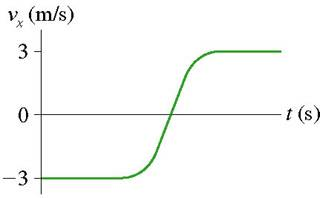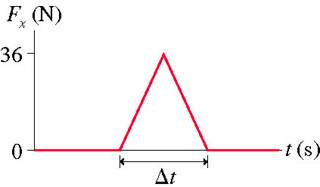# Impulse momentum theorum with a spring

## Homework Statement

A 700 g air-track glider collides with a spring at one end of the track. The figure shows the glider's velocity and the force exerted on the glider by the spring.

How long is the glider in contact with the spring?## Homework Equations

F(t_1-t_2)=mv_2-mv_1

## The Attempt at a Solution

I have the force as 36N, v_1 as -3, v_2 as 3.
When I plug this into the equation I get
change in t = (700*3-700*(-3))/36=116.6

I know this has to be wrong but I'm confused which variable I have wrong. Maybe the force?

Last edited:

## Answers and Replies

hage567
Homework Helper
You need to convert 700 g to kg. Other than that, I think your solution is correct.

change in t = ((.7*3)-(.7*-3))/36 = .116 s is also wrong.

hage567
Homework Helper
Sorry, you need to use the average force. The 36 N is the peak force.

I'm still stuck on this one. I know that i can calculate avg force by impulse / change in time, but I'm not sure how to find avg force in this situation.

In case anyone else has a similar problem I'll explain how I found the answer. I found the area of the triangle with. A = .5 * (36, force max) * change in t. A is equal to weight in kg * v_1 - (weight in kg * v_2). Therefore 4.2 = .5 (36) * change in t.

In case anyone else has a similar problem I'll explain how I found the answer. I found the area of the triangle with. A = .5 * (36, force max) * change in t. A is equal to weight in kg * v_1 - (weight in kg * v_2). Therefore 4.2 = .5 (36) * change in t.

I know this is an old message but this poster states he solved the problem by finding.

(simply restating correct solution posted in quotes here)

A=area

A=.5(Fmax)Δt

A=mv1-mv2

I think? I don't understand what equations the poster was trying to write out.

Wouldn't the change in momentum be zero since the final and initial speeds are equal?

Or would it be double the current momentum because of the change in direction?